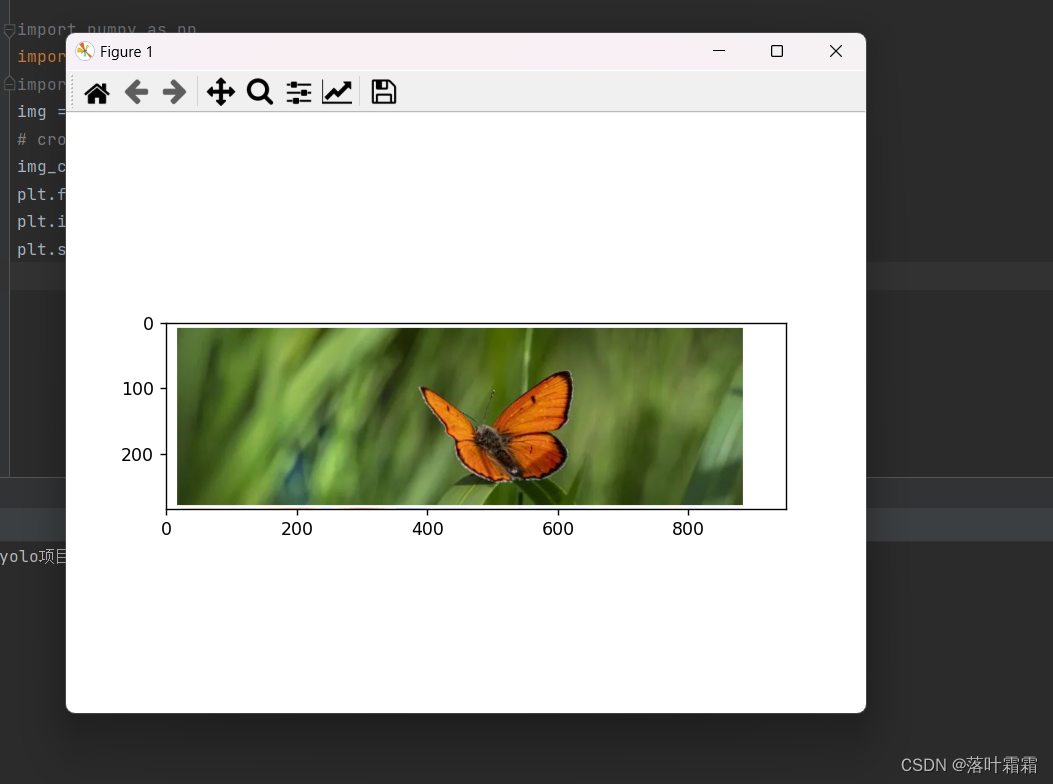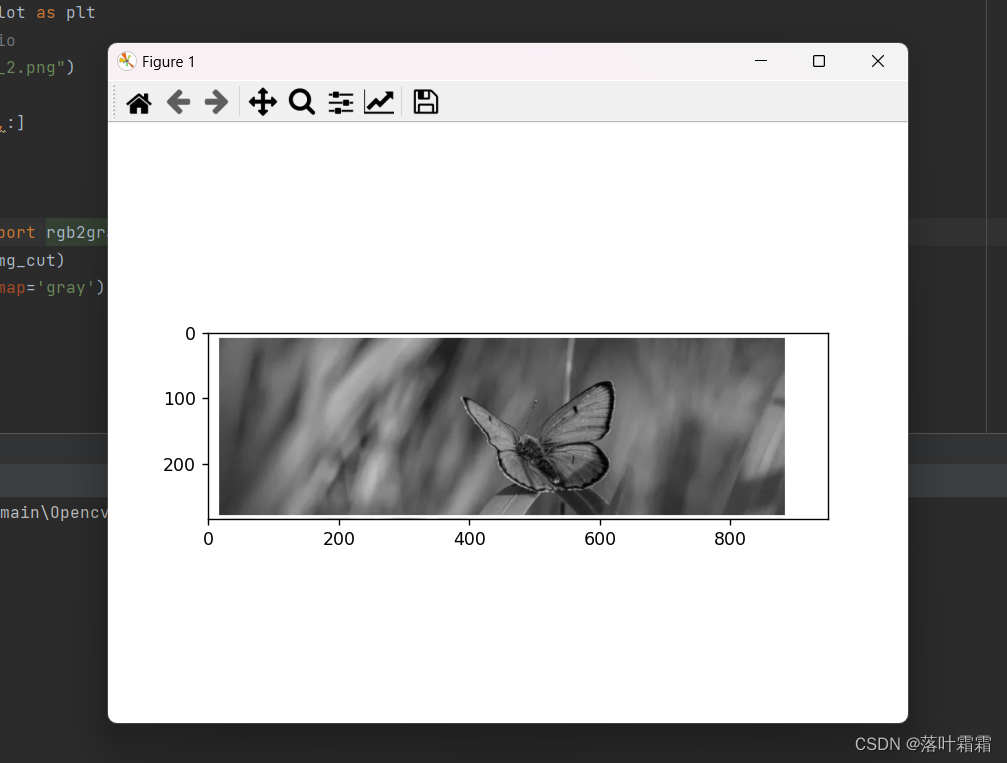# 【OpenCV实现图像：OpenCV利用Python创作热力图】

• 概要
• 读取图像
• 图像灰度化
• **像素化效果**
• 小结

### 读取图像

``import numpy as npimport matplotlib.pyplot as pltimport skimage.io as ioimg = plt.imread("img_2.png")# cropimg_cut = img[0:400,:,:]plt.figure()plt.imshow(img_cut)plt.show()``### 图像灰度化

``import numpy as npimport matplotlib.pyplot as pltimport skimage.io as ioimg = plt.imread("img_2.png")# cropimg_cut = img[0:400,:,:]plt.figure()plt.imshow(img_cut)plt.show()from skimage.color import rgb2graygray_img = rgb2gray(img_cut)plt.imshow(gray_img,cmap='gray')plt.show()``### 像素化效果

``from PIL import Imagedef pixelate(image_path, pixel_size): # 打开图片 img = Image.open(image_path)# 获取图片的宽度和高度 width, height = img.size # 计算每个像素块的大小 pixel_size = max(1, pixel_size) block_size =(width // pixel_size, height // pixel_size)# 缩小图像，然后放大回原始大小，实现像素化效果 img = img.resize(block_size, resample=Image.NEAREST) img = img.resize((width, height), resample=Image.NEAREST)# 保存处理后的图像 output_path ="pixelated_" + str(pixel_size) + "_" + image_path img.save(output_path)# 使用例子image_path ="img_2.png"pixel_size =10pixelate(image_path, pixel_size)````pip install seaborn``

``import seaborn as snsimport matplotlib.pyplot as pltfrom PIL import Imageimport numpy as npdef heatmap_from_image(image_path, pixel_size): # 打开处理后的图片 img = Image.open("pixelated_" + str(pixel_size) + "_" + image_path)# 将图像转为NumPy数组 img_array = np.array(img)# 创建热力图 plt.figure(figsize=(10, 8)) sns.heatmap(img_array[:, :, 0], cmap="viridis", cbar=False)# 设置图像标题和坐标轴标签 plt.title("Heatmap from Pixelated Image") plt.xlabel("X-axis") plt.ylabel("Y-axis")# 显示热力图 plt.show()# 使用例子heatmap_from_image("img_2.png", 10)``

Seaborn库提供了丰富的调色板选项，允许您自定义热力图的颜色样式。在上面的代码中，我们使用了viridis调色板，如果您想尝试其他调色板，只需修改cmap参数即可。例如，我们将代码中的"cmap"参数改为"coolwarm"，效果如下：

``import seaborn as snsimport matplotlib.pyplot as pltfrom PIL import Imageimport numpy as npdef heatmap_with_custom_palette(image_path, pixel_size, palette_name): # 打开处理后的图片 img = Image.open("pixelated_" + str(pixel_size) + "_" + image_path)# 将图像转为NumPy数组 img_array = np.array(img)# 创建热力图 plt.figure(figsize=(10, 8)) sns.heatmap(img_array[:, :, 0], cmap=palette_name, cbar=False)# 设置图像标题和坐标轴标签 plt.title(f"Heatmap with {palette_name} Palette") plt.xlabel("X-axis") plt.ylabel("Y-axis")# 显示热力图 plt.show()# 使用例子heatmap_with_custom_palette("img_2.png", 10, "coolwarm")``